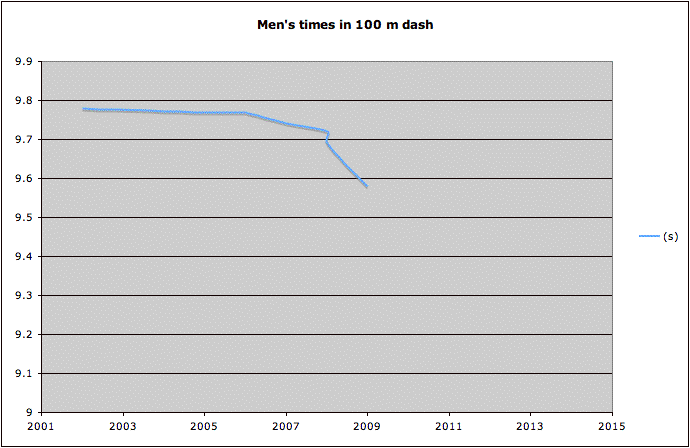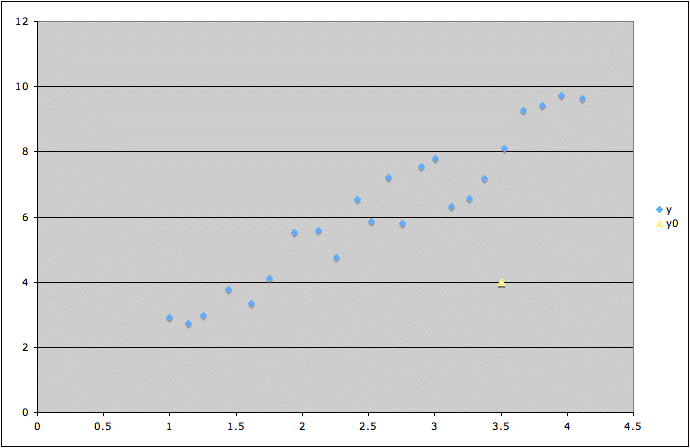# Extrapolation Beyond the Range of the Data

Example: The following graph shows a curve fitted to men's best times in the 100 m dash for 2001 through 2009.If the trend for years before 2006 had been used to predict the times for 2008 and 2009, the estimate would be noticeably over the actual times. If the trend for 2008 to 2009 is used to predict the time for 2013, we would have some very amazing times.

In some instances, the purpose of a study is indeed to predict what will happen next month or next year, based on recent data. The researcher has to do the best they can. But predicting farther in the future leads to more uncertainty.

A similar problem occurs, but is not so easy to detect, when considering more variables.

Example: Consider a study whose purpose is to try to predict variable z in terms of variables x and y. Suppose the graph below shows the plot of y versus x values for the data in blue,  but it is desired to predict z for a case where the x and y values are shown by the yellow point. This prediction could be considered extrapolation beyond the range of the data, since the blue data points all lie within a roughly elliptical region, but the yellow point lies noticeably outside that region. (Note that in this example, we can detect that the yellow point lies outside the range of the data simply by graphing the data. In situations with more variables, the Mahalanobis distance is sometimes appropriate to detect possible extrapolation beyond the range of the data.)